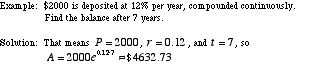# continuous compounding

Continuously Compounded Interest

Interest that is, hypothetically, computed and added to the balance of an account every instant. This is not actually possible, but continuous compounding is well-defined nevertheless as the upper bound of "regular" compound interest. The formula, given below, is sometimes called the shampoo formula (Pert®).

Note: This same formula can be used for exponential growth and exponential decay.